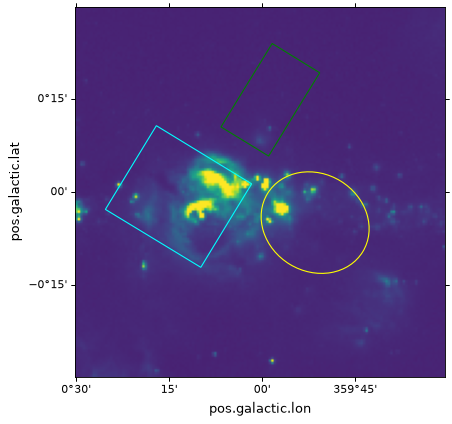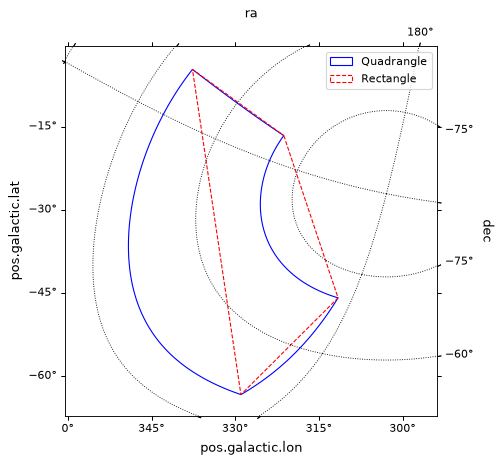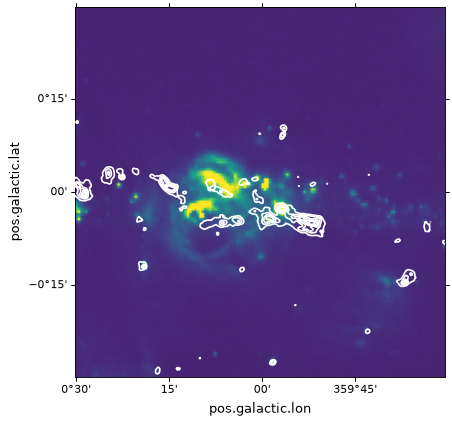# Overplotting markers and artists¶

For the example in the following page we start from the example introduced in Initializing axes with world coordinates.

## Pixel coordinates¶

Apart from the handling of the ticks, tick labels, and grid lines, the WCSAxes class behaves like a normal Matplotlib Axes instance, and methods such as imshow(), contour(), plot(), scatter(), and so on will work and plot the data in pixel coordinates by default.

In the following example, the scatter markers and the rectangle will be plotted in pixel coordinates:

# The following line makes it so that the zoom level no longer changes,
# otherwise Matplotlib has a tendency to zoom out when adding overlays.
ax.set_autoscale_on(False)

# Add a rectangle with bottom left corner at pixel position (30, 50) with a
# width and height of 60 and 50 pixels respectively.
from matplotlib.patches import Rectangle
r = Rectangle((30., 50.), 60., 50., edgecolor='yellow', facecolor='none')

# Add three markers at (40, 30), (100, 130), and (130, 60). The facecolor is
# a transparent white (0.5 is the alpha value).
ax.scatter([40, 100, 130], [30, 130, 60], s=100, edgecolor='white', facecolor=(1, 1, 1, 0.5))


(png, svg, pdf)## World coordinates¶

All such Matplotlib commands allow a transform= argument to be passed, which will transform the input from world to pixel coordinates before it is passed to Matplotlib and plotted. For instance:

ax.scatter(..., transform=...)


will take the values passed to scatter() and will transform them using the transformation passed to transform=, in order to end up with the final pixel coordinates.

The WCSAxes class includes a get_transform() method that can be used to get the appropriate transformation object to convert from various world coordinate systems to the final pixel coordinate system required by Matplotlib. The get_transform() method can take a number of different inputs, which are described in this and subsequent sections. The two simplest inputs to this method are 'world' and 'pixel'.

For example, if your WCS defines an image where the coordinate system consists of an angle in degrees and a wavelength in nanometers, you can do:

ax.scatter(, [3.2], transform=ax.get_transform('world'))


to plot a marker at (34deg, 3.2nm).

Using ax.get_transform('pixel') is equivalent to not using any transformation at all (and things then behave as described in the Pixel coordinates section).

## Celestial coordinates¶

For the special case where the WCS represents celestial coordinates, a number of other inputs can be passed to get_transform(). These are:

• 'fk4': B1950 FK4 equatorial coordinates

• 'fk5': J2000 FK5 equatorial coordinates

• 'icrs': ICRS equatorial coordinates

• 'galactic': Galactic coordinates

In addition, any valid astropy.coordinates coordinate frame can be passed.

For example, you can add markers with positions defined in the FK5 system using:

ax.scatter(266.78238, -28.769255, transform=ax.get_transform('fk5'), s=300,
edgecolor='white', facecolor='none')


(png, svg, pdf)In the case of scatter() and plot(), the positions of the center of the markers is transformed, but the markers themselves are drawn in the frame of reference of the image, which means that they will not look distorted.

## Patches/shapes/lines¶

Transformations can also be passed to Matplotlib patches. For example, we can use the get_transform() method above to plot a rectangle in FK5 equatorial coordinates:

r = Rectangle((266.0, -28.9), 0.3, 0.15, edgecolor='green', facecolor='none',
transform=ax.get_transform('fk5'))


(png, svg, pdf)In this case, the rectangle will be plotted at FK5 J2000 coordinates (266deg, -28.9deg). However, it is very important to note that while the height will indeed be 0.15 degrees, the width will not strictly represent 0.3 degrees on the sky, but an interval of 0.3 degrees in longitude (which, depending on the latitude, will represent a different angle on the sky). In other words, if the width and height are set to the same value, the resulting polygon will not be a square, and the same applies to the Circle patch, which will not actually produce a circle:

from matplotlib.patches import Circle

r = Rectangle((266.4, -28.9), 0.3, 0.3, edgecolor='cyan', facecolor='none',
transform=ax.get_transform('fk5'))

c = Circle((266.4, -29.1), 0.15, edgecolor='yellow', facecolor='none',
transform=ax.get_transform('fk5'))


(png, svg, pdf)Important

If what you are interested is simply plotting circles around sources to highlight them, then we recommend using scatter(), since for the circular marker (the default), the circles will be guaranteed to be circles in the plot, and only the position of the center is transformed.

To plot ‘true’ spherical circles, see the Spherical patches section.

## Contours¶

Overplotting contours is also simple using the get_transform() method. For contours, get_transform() should be given the WCS of the image to plot the contours for:

filename = get_pkg_data_filename('galactic_center/gc_bolocam_gps.fits')
hdu = fits.open(filename)
levels=[1,2,3,4,5,6], colors='white')


(png, svg, pdf)## Spherical patches¶

In the case where you are making a plot of a celestial image, and want to plot a circle that represents the area within a certain angle of a longitude/latitude, the Circle patch is not appropriate, since it will result in a distorted shape (because longitude is not the same as the angle on the sky). For this use case, you can instead use SphericalCircle, which takes a tuple of Quantity as the input, and a Quantity as the radius:

from astropy import units as u
from astropy.visualization.wcsaxes import SphericalCircle

r = SphericalCircle((266.4 * u.deg, -29.1 * u.deg), 0.15 * u.degree,
edgecolor='yellow', facecolor='none',
transform=ax.get_transform('fk5'))